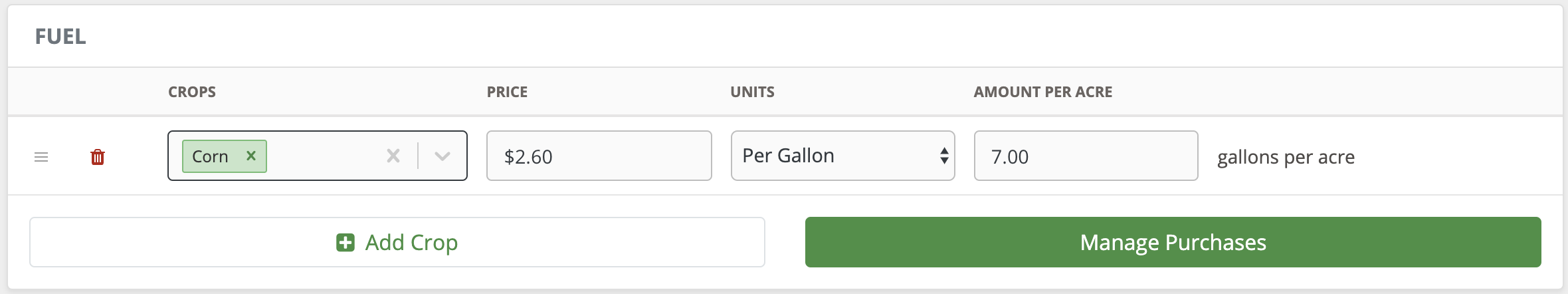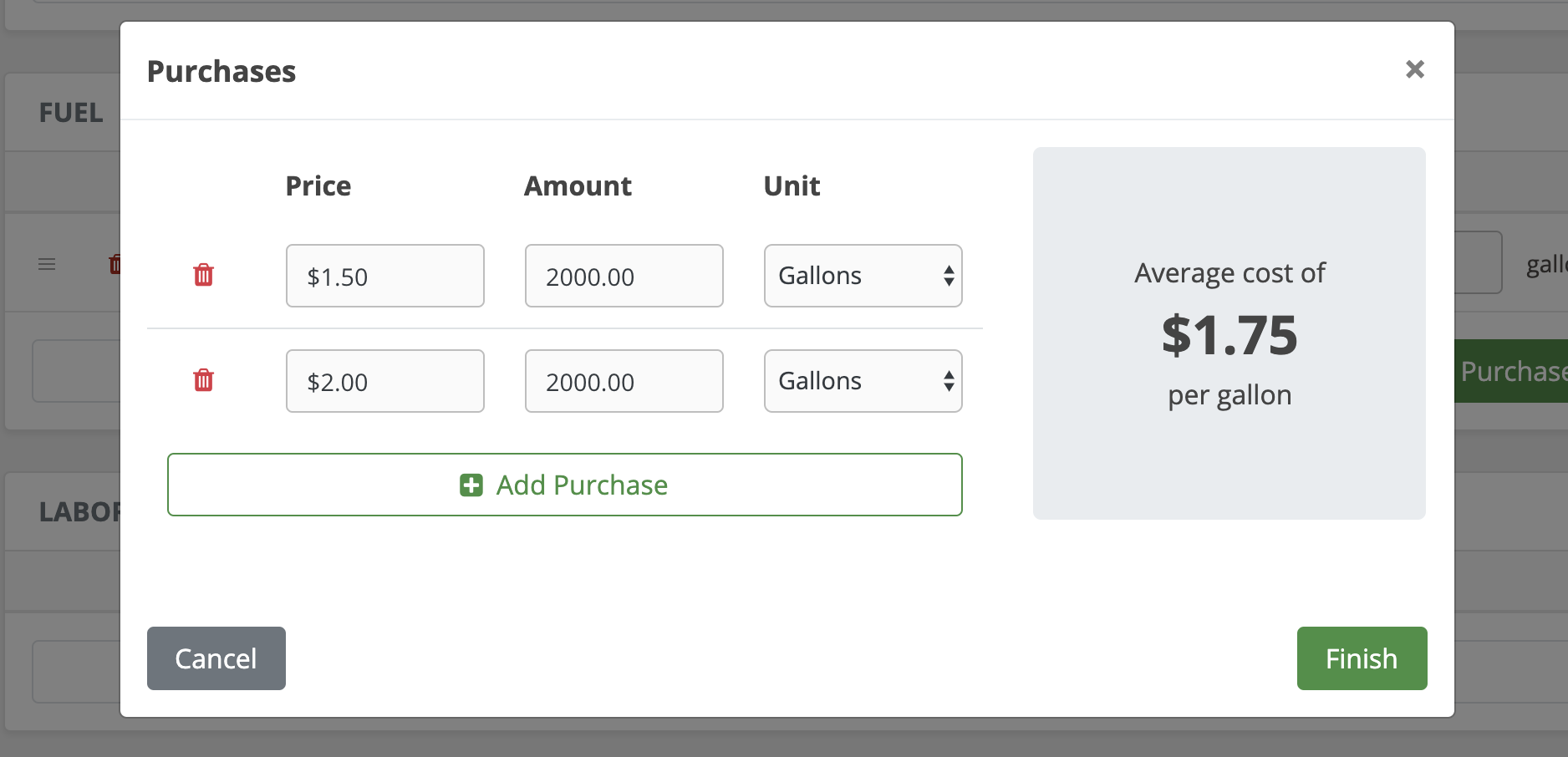# How are fuel costs calculated?

Per gallon fuel costs are taken from the Inputs tab and applied to your fields based on the per acre usage rates.

The per acre price is determined by multiplying the price times the amount applied per acre. So if there is a fuel input applied to "Corn", with a "Price" of \$2.60 per gallon and 7.00 gallons per acre, the "Corn" fields will have a per acre fuel cost of \$18.20 (\$2.60 per gallon x 7.00 gallons per acre).The purchases you add on the Inputs tab are used to determine the average fuel price (per gallon or liter).

For example, let's say you enter 2,000 gallons of fuel at \$1.50 and 2,000 gallons at \$2.00.  Your average per gallon fuel price will then be a rate of \$1.75/gallon.The order of your fuel purchases doesn't matter.  The software simply uses an average price.